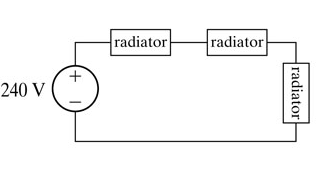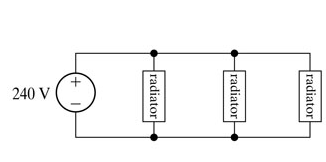Create an Accountfigure 1figure 2

1)Using the wiring diagram shown in (Figure 1) , calculate the total power for the three radiators.

2) Compare the total radiator power in this configuration with the total radiator power (p?total) in the configuration shown in (Figure 2).

Jul 27 2020 View more View LessSubscribe To Get Solution